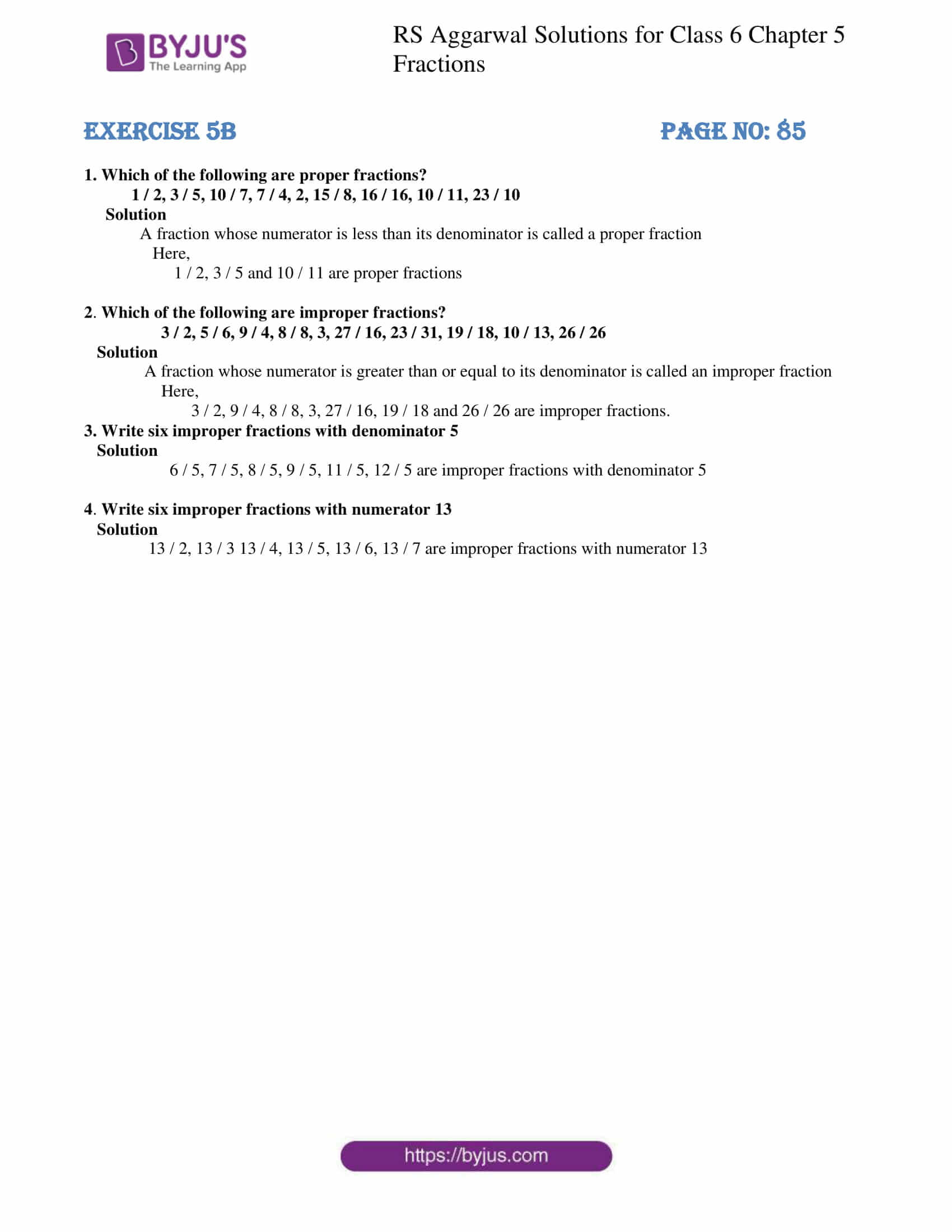# RS Aggarwal Solutions for Class 6 Maths Chapter 5 Fractions Exercise 5B

RS Aggarwal Solutions for Class 6 Maths Chapter 5 Fractions Exercise 5B are available here with free download option. Exercise 5B of RS Aggarwal Solutions consists of questions based on proper, improper and mixed fractions along with the steps which are followed in converting them. A fraction whose numerator is less than its denominator is called a proper fraction. A fraction whose numerator is greater than or equal to its denominator is called an improper fraction. A combination of a whole number and a proper fraction is called a mixed fraction. Students who wish to study these topics further are advised to go through RS Aggarwal Solutions for Class 6 Chapter 5 Fractions.

## Download PDF of RS Aggarwal Solutions for Class 6 Chapter 5 Fractions Exercise 5B### Access answers to Maths RS Aggarwal Solutions for Class 6 Chapter 5 Fractions Exercise 5B

1. Which of the following are proper fractions?

1 / 2, 3 / 5, 10 / 7, 7 / 4, 2, 15 / 8, 16 / 16, 10 / 11, 23 / 10

Solution

A fraction whose numerator is less than its denominator is called a proper fraction

Here,

1 / 2, 3 / 5 and 10 / 11 are proper fractions

2. Which of the following are improper fractions?

3 / 2, 5 / 6, 9 / 4, 8 / 8, 3, 27 / 16, 23 / 31, 19 / 18, 10 / 13, 26 / 26

Solution

A fraction whose numerator is greater than or equal to its denominator is called an improper fraction

Here,

3 / 2, 9 / 4, 8 / 8, 3, 27 / 16, 19 / 18 and 26 / 26 are improper fractions.

3. Write six improper fractions with denominator 5

Solution

6 / 5, 7 / 5, 8 / 5, 9 / 5, 11 / 5, 12 / 5 are improper fractions with denominator 5

4. Write six improper fractions with numerator 13

Solution

13 / 2, 13 / 3 13 / 4, 13 / 5, 13 / 6, 13 / 7 are improper fractions with numerator 13